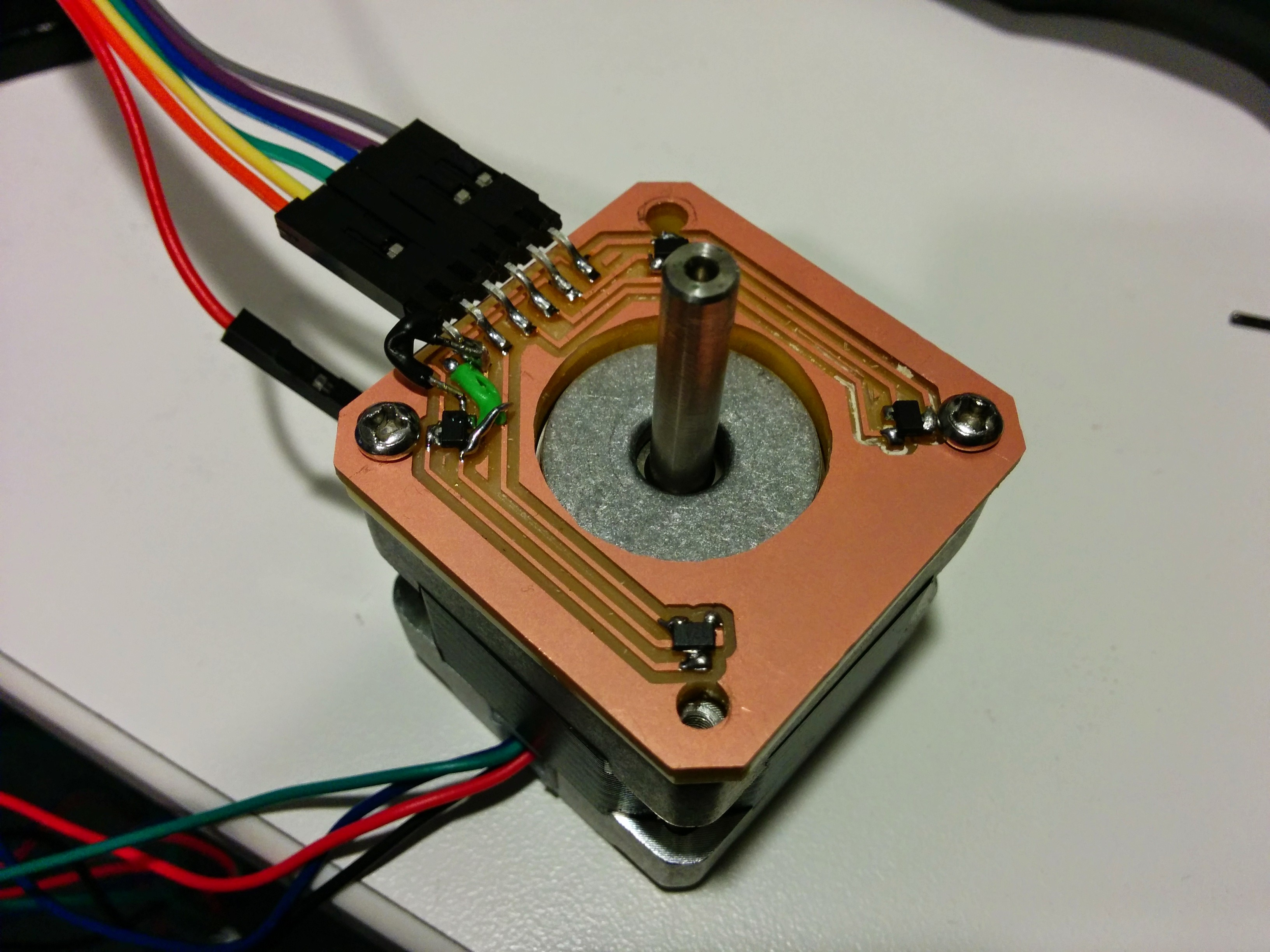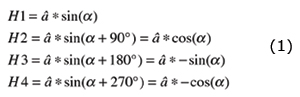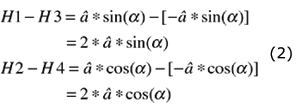For my plotbot I've decided to build a magnetic rotary encoder that can sense a precise angle.

The idea: use 4 hall sensors to magically calculate the exact orientation of the wheel. I was assisted by a sensormag article : "Understanding Integrated Hall Effect Rotary Encoders"

#### Magnetized Wheel

I started by creating a wheel that has a diametric polarity (one direction is positive and the other is negative).#### Circuitry

The 4 hall sensors are positioned in the exact distance from the center, offset by 90 degrees from each other.#### Test code

The following code loops over 200 steps which equal 360 degrees. after each step It takes a measurement from all the hall sensors. The output is a simple CSV.

``````void loop() {

if (Serial.available()>0) {
on = !on;
a = 0;
}

if (on && a<200) {
Serial.print(a++);
Serial.print(",");
Serial.print(",");
Serial.print(",");
Serial.print(",");
Serial.print("\n");
digitalWrite(stp, HIGH);
delay(10);
digitalWrite(stp, LOW);
delay(10);
} else {
delay(20);
}
}``````

#### Run

And on the serial monitor:

``````step, A0, A1, A2, A3
0,513,524,528,510
1,511,526,526,511
2,511,527,526,509
3,513,525,528,508
....
....
197,512,524,526,511
198,511,525,526,512
199,513,524,528,509
``````

#### Math

I imported the above csv into google sheets, replaced steps with angle (steps*1.8) and plotted the table.

I performed basic calibration by offsetting each value to by it's neutral value and normalizing the values between min and max amplitude.Given that we know how all these functions behave I could now calculate all the angles (with an offset because I didn't start from an angle of 0)Comparing my calculated angle steps to the original angle steps show a variance of 4.702 degrees and a standard deviation of 2.168 degrees.

This is not good enough, I then tried to improve this by using more precise analog reads. This is possible with an arduino uno using oversampling. This is a very wasteful procedure so I'll only use it for testing.

Using an analog oversampling library I recreated the experiment with 14 bit precision and averaged 3 measurements per step.

Going through the entire process again I achieve a variance of 0.130 degrees and standard deviation of 0.361 degrees. Not so bad!